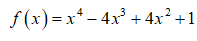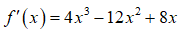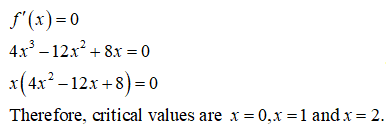# For the function, find all critical numbers and then use the second-derivative test to determine whether the function has a relative maximum or minimum at each critical number. (Enter your answers as a comma-separated list. If an answer does not exist, enter DNE.)f(x) = x4 − 4x3 + 4x2 + 1 Relative Maxima x=Relative Minima  x=

Question
2 views

For the function, find all critical numbers and then use the second-derivative test to determine whether the function has a relative maximum or minimum at each critical number. (Enter your answers as a comma-separated list. If an answer does not exist, enter DNE.)

f(x) = x4 − 4x3 + 4x2 + 1

Relative Maxima x=
Relative Minima  x=
check_circle

Step 1

The given function is,Step 2

Obtain the first derivative of the function.Step 3

The critical values for the function is obtained as follows....

### Want to see the full answer?

See Solution

#### Want to see this answer and more?

Solutions are written by subject experts who are available 24/7. Questions are typically answered within 1 hour.*

See Solution
*Response times may vary by subject and question.
Tagged in

### Calculus